# forecast

Forecast sample paths from threshold-switching dynamic regression model

## Syntax

``YF = forecast(Mdl,Y,numPeriods)``
``YF = forecast(Mdl,Y,numPeriods,Name,Value)``
``[YF,EstCov] = forecast(___)``

## Description

example

````YF = forecast(Mdl,Y,numPeriods)` returns optimal one-step-ahead point forecasts `YF` of a fully specified threshold-switching dynamic regression model `Mdl` over a forecast horizon of length `numPeriods`. The forecasted responses represent the continuation of the response data `Y`.```

example

````YF = forecast(Mdl,Y,numPeriods,Name,Value)` uses additional options specified by one or more name-value arguments. For example, `forecast(Mdl,Y,10,Type="exogenous",Z=z)` specifies exogenous-type forecasts and forecast-period, exogenous threshold variable data `z`.```

example

````[YF,EstCov] = forecast(___)` returns simulation-based forecasts `YF` and corresponding forecast error covariances `EstCov`, using any of the input argument combinations in the previous syntaxes. ```

## Examples

collapse all

This example shows how to compute iterative point forecasts of the conditional mean of a self-exciting threshold autoregressive (SETAR) model. Specify all parameter values (this example uses arbitrary values).

Create Fully Specified Model for DGP

Create a discrete threshold transition at level 0.

```t = 0; tt = threshold(t)```
```tt = threshold with properties: Type: 'discrete' Levels: 0 Rates: [] StateNames: ["1" "2"] NumStates: 2 ```

`tt` is a fully specified `threshold` object that describes the switching mechanism of the threshold-switching model.

Assume the following univariate models describe the response process of the system:

• State 1: ${\mathit{y}}_{\mathit{t}}=-1+0.6{\mathit{y}}_{\mathit{t}-1}+{\epsilon }_{\mathit{t}}$.

• State 2: ${\mathit{y}}_{\mathit{t}}=1+0.6{\mathit{y}}_{\mathit{t}-1}+{\epsilon }_{\mathit{t}}$.

• ${\epsilon }_{\mathit{t}}\sim Ν\left(0,0.5\right)$.

For each regime, use `arima` to create an AR model that describes the response process within the regime.

```c1 = -1; c2 = 1; ar = 0.6; mdl1 = arima(Constant=c1,AR=ar)```
```mdl1 = arima with properties: Description: "ARIMA(1,0,0) Model (Gaussian Distribution)" Distribution: Name = "Gaussian" P: 1 D: 0 Q: 0 Constant: -1 AR: {0.6} at lag  SAR: {} MA: {} SMA: {} Seasonality: 0 Beta: [1×0] Variance: NaN ```
`mdl2 = arima(Constant=c2,AR=ar)`
```mdl2 = arima with properties: Description: "ARIMA(1,0,0) Model (Gaussian Distribution)" Distribution: Name = "Gaussian" P: 1 D: 0 Q: 0 Constant: 1 AR: {0.6} at lag  SAR: {} MA: {} SMA: {} Seasonality: 0 Beta: [1×0] Variance: NaN ```

`mdl1` and `mdl2` are effectively, fully specified `arima` objects. The innovations variances of the submodels are unspecified; the tsVAR object specifies the model-wide innovations variance.

Store the submodels in a vector with order corresponding to the regimes in `tt.StateNames`.

`mdl = [mdl1; mdl2];`

Use `tsVAR` to create a TAR model from the switching mechanism `tt` and the state-specific submodels `mdl`. Specify a model-wide innovations variance of 0.5.

`Mdl = tsVAR(tt,mdl,Covariance=0.5)`
```Mdl = tsVAR with properties: Switch: [1x1 threshold] Submodels: [2x1 varm] NumStates: 2 NumSeries: 1 StateNames: ["1" "2"] SeriesNames: "1" Covariance: 0.5000 ```
`Mdl.Submodels(2)`
```ans = varm with properties: Description: "AR-Stationary 1-Dimensional VAR(1) Model" SeriesNames: "Y1" NumSeries: 1 P: 1 Constant: 1 AR: {0.6} at lag  Trend: 0 Beta: [1×0 matrix] Covariance: NaN ```

`Mdl` is a fully specified `tsVAR` object representing a univariate two-state TAR model. `tsVAR` stores specified `arima` submodels as `varm` objects.

Simulate Response Data from DGP

`forecast` requires enough data before the forecast horizon to initialize the model. Simulate 120 observations from the DGP.

```rng(1); % For reproducibility y = simulate(Mdl,120);```

y is a 120-by-1 random path of responses. The default options of `simulate`, and all `tsVAR` object functions, specify that the threshold variable is ${\mathit{y}}_{\mathit{t}-1}$.

Compute Optimal Point Forecasts

Treat the first 100 observations of the simulated response data as the presample for the forecast, and treat the last 10 observations as a holdout sample.

```idx0 = 1:100; idx1 = 101:120; y0 = y(idx0); y1 = y(idx1);```

Compute 1- through 20-step-ahead optimal point forecasts from the model.

`yf = forecast(Mdl,y0,20);`

`yf` is a 20-by-1 vector of optimal point forecasts.

Plot the simulated response data and forecasts.

```figure hold on plot(idx0,y0,'b'); h = plot(idx1,y1,'b--'); h1 = plot(idx1,yf,'r'); yfill = [ylim fliplr(ylim)]; xfill = [idx0(end) idx0(end) idx1(end) idx1(end)]; fill(xfill,yfill,'k','FaceAlpha',0.05) legend([h h1],["Actual" "Optimal"],'Location','NorthWest') title('Forecasts') hold off````forecast` must use Monte Carlo methods to estimate forecast error variances. Therefore, when `simulate` returns forecast error variances, it uses Monte Carlo methods to compute point forecasts.

Create the SETAR model in Compute Optimal Point Forecasts.

```t = 0; tt = threshold(t); c1 = -1; c2 = 1; ar = 0.6; mdl1 = arima(Constant=c1,AR=ar); mdl2 = arima(Constant=c2,AR=ar); mdl = [mdl1; mdl2]; Mdl = tsVAR(tt,mdl,Covariance=0.5);```

`forecast` requires enough data before the forecast horizon to initialize the model. Simulate 120 observations from the DGP.

```rng(100); % For reproducibility fh = 20; y = simulate(Mdl,100+fh);```

Treat the first 100 observations of the simulated response data as the presample for the forecast, and treat the last 20 observations as a holdout sample.

```idx0 = 1:100; idx1 = 101:(100 + fh); y0 = y(idx0); y1 = y(idx1);```

Compute 1- through 20-step-ahead optimal point forecasts from the model.

`yf1 = forecast(Mdl,y0,fh);`

`yf1` is a 20-by-1 vector of optimal point forecasts.

Compute 1- through 20-step-ahead Monte Carlo point forecasts by returning the estimated forecast error variances.

`[yf2,estVar] = forecast(Mdl,y0,fh);`

`yf2` is a 20-by-1 vector of Monte Carlo point forecasts. `estVAR` is a 20-by-1 vector of corresponding estimated forecast error variances.

Plot the simulated response data, forecasts, and 95% forecast intervals using the Monte Carlo estimates.

```figure hold on plot(idx0,y0,'b'); h = plot(idx1,y1,'b--'); h1 = plot(idx1,yf1,'r'); h2 = plot(idx1,yf2,'m'); ciu = yf2 + 1.96*sqrt(estVar); % Upper 95% confidence level cil = yf2 - 1.96*sqrt(estVar); % Lower 95% confidence level plot(idx1,ciu,'m-.'); plot(idx1,cil,'m-.'); yfill = [ylim,fliplr(ylim)]; xfill = [idx0(end) idx0(end) idx1(end) idx1(end)]; fill(xfill,yfill,'k','FaceAlpha',0.05) legend([h h1 h2],["Actual" "Optimal" "Estimated"],... 'Location',"NorthWest") title("Point and Interval Forecasts") hold off```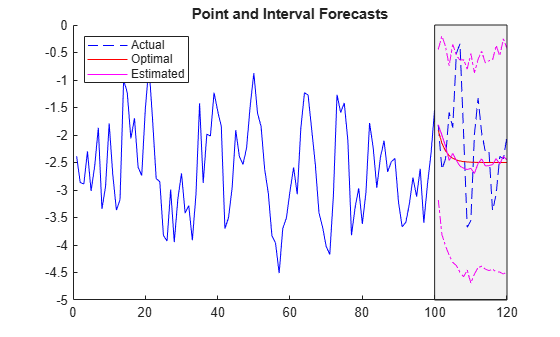When `forecast` performs Monte Carlo methods to estimate point forecasts and forecast error covariances, you can adjust the number of paths to sample by specifying the `NumPaths` option.

Create the SETAR model in Compute Optimal Point Forecasts.

```t = 0; tt = threshold(t); c1 = -1; c2 = 1; ar = 0.6; mdl1 = arima(Constant=c1,AR=ar); mdl2 = arima(Constant=c2,AR=ar); mdl = [mdl1; mdl2]; Mdl = tsVAR(tt,mdl,Covariance=0.5);```

`forecast` requires enough data before the forecast horizon to initialize the model. Simulate 120 observations from the DGP.

```rng(100); % For reproducibility fh = 20; y = simulate(Mdl,100+fh);```

Treat the first 100 observations of the simulated response data as the presample for the forecast, and treat the last 20 observations as a holdout sample.

```idx0 = 1:100; idx1 = 101:(100 + fh); y0 = y(idx0); y1 = y(idx1);```

Compute 1- through 20-step-ahead optimal point forecasts from the model.

`yf1 = forecast(Mdl,y0,fh);`

Compute 1- through 20-step-ahead Monte Carlo point forecasts by returning the estimated forecast error variances. Specify a Monte Carlo sample of 1000 paths.

`[yf2,estVar] = forecast(Mdl,y0,fh,NumPaths=1000);`

`yf2` is a 20-by-1 vector of Monte Carlo point forecasts. `estVAR` is a 20-by-1 vector of corresponding estimated forecast error variances.

Plot the simulated response data, forecasts, and 95% forecast intervals using the Monte Carlo estimates.

```figure hold on plot(idx0,y0,'b'); h = plot(idx1,y1,'b--'); h1 = plot(idx1,yf1,'r'); h2 = plot(idx1,yf2,'m'); ciu = yf2 + 1.96*sqrt(estVar); % Upper 95% confidence level cil = yf2 - 1.96*sqrt(estVar); % Lower 95% confidence level plot(idx1,ciu,'m-.'); plot(idx1,cil,'m-.'); yfill = [ylim,fliplr(ylim)]; xfill = [idx0(end) idx0(end) idx1(end) idx1(end)]; fill(xfill,yfill,'k',FaceAlpha=0.05) legend([h h1 h2],["Actual" "Optimal" "Estimated"],Location="NorthWest") title("Point and Interval Forecasts") hold off```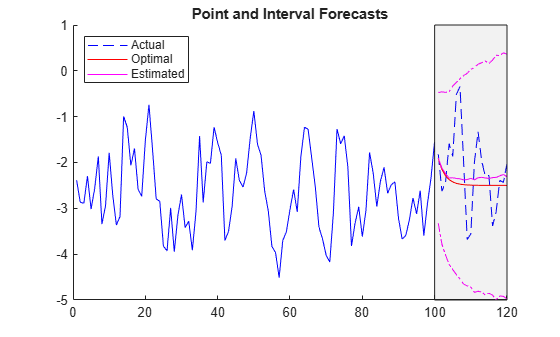Consider a logistic threshold-switching model (LSTAR) for the real US GDP growth rate, where each submodel is AR(4) and the threshold variable is the unemployment growth rate.

Create a partially specified threshold transition for the unemployment growth rate. Specify the logistic transition function, and an unknown, estimable mid-level and rate. Label the states `"Contraction"` and `"Expansion"`.

```tt = threshold(NaN,Type="logistic",Rates=NaN,... StateNames=["Contraction" "Expansion"]);```

`tt` is a partially specified `threshold` object, and it is agnostic of the variable and data it represents.

Create a threshold-switching model for the real US GDP growth rate.

```p = 4; mdl = [arima(ARLags=p); arima(ARLags=p)]; Mdl = tsVAR(tt,mdl);```

`Mdl` is a partially specified `tsVAR` object specifying the sturcture of the model and which parameters are estimable.

Load the quarterly US macroeconomic data set `Data_USEconModel`. Remove leading missing values in the series. Compute the real GDP percent growth and unemployment growth.

```load Data_USEconModel DataTable = rmmissing(DataTable,DataVariables=["GDP" "GDPDEF" "UNRATE"]); RGDP = DataTable.GDP./DataTable.GDPDEF; rRGDP = price2ret(RGDP); % Response data gUNRATE = diff(DataTable.UNRATE); % Exogenous thresold data dts = DataTable.Time(2:end); T = numel(dts);```

Hold out the following sets from estimation:

• The first four observations as a presample for estimation.

• The final 25% as a forecast horizon to compare with the forecasts.

```fh = ceil(0.25*T); idxPre = 1:p; idxEst = (idxPre(end)+1):(T-fh); idxF = (T-fh+1):T;```

To initialize the estimation procedure, fully specify a threshold transition that has the same structure as `tt`, but set the mid-level to 0 and a rate of 1 (the default).

`tt0 = threshold(0,Type=tt.Type);`

Fit the LSTAR model to the estimation period of the US GDP growth rate series. Specify the following parameters:

• Set `Y0` to the responses before the estimation period to initialize the AR submodel components.

• Set `Type` to `"exogenous"` to characterize the threshold variable.

• Set `Z` to the threshold variable data `gUNRATE` in the estimation period.

```EstMdl = estimate(Mdl,tt0,rRGDP(idxEst),Y0=rRGDP(idxPre), ... Type="exogenous",Z=gUNRATE(idxEst));```

Forecast the real GDP growth rate series into the forecast horizon. Initialize the forecasts by specifying all responses in the estimation period (`forecast` uses only the latest, required observations). Characterize the threshold variable and provide its data in the forecast horizon.

`yF = forecast(EstMdl,rRGDP(idxEst),fh,Type="exogenous",Z=gUNRATE(idxF));`

Plot the real GDP growth rate series with the forecasts.

```figure hold on h1 = plot(dts(idxEst),rRGDP(idxEst),'b'); h2 = plot(dts(idxF),rRGDP(idxF),'b--'); h3 = plot(dts(idxF),yF,'r'); yfill = [ylim,fliplr(ylim)]; xfill = dts([idxEst(end) idxEst(end) idxF(end) idxF(end)]); fill(xfill,yfill,'k',FaceAlpha=0.05) legend([h1 h2 h3],["Data (estimation)" "Data (hold out)" "Forecasts"],... Location="NorthWest") title("Real GDP Growth Rate Point Forecasts") hold off```Consider the following 2-D LSETAR model.

• State 1, `"Low"`: ${y}_{t}=\left[\begin{array}{c}1\\ -1\end{array}\right]+{\epsilon }_{1,t},$ where ${\epsilon }_{1,t}\sim N\left(\left[\begin{array}{c}0\\ 0\end{array}\right],\left[\begin{array}{cc}1& -0.1\\ -0.1& 1\end{array}\right]\right).$

• State 2 , `"Med"`: ${y}_{t}=\left[\begin{array}{c}2\\ -2\end{array}\right]+\left[\begin{array}{cc}0.5& 0.1\\ 0.5& 0.5\end{array}\right]{y}_{t-1}+{\epsilon }_{2,t},$ where ${\epsilon }_{2,t}\sim N\left(\left[\begin{array}{c}0\\ 0\end{array}\right],\left[\begin{array}{cc}2& -0.2\\ -0.2& 2\end{array}\right]\right).$

• State 3, `"High"`: ${y}_{t}=\left[\begin{array}{c}3\\ -3\end{array}\right]+\left[\begin{array}{cc}0.25& 0\\ 0& 0\end{array}\right]{y}_{t-1}+\left[\begin{array}{cc}0& 0\\ 0.25& 0\end{array}\right]{y}_{t-2}+{\epsilon }_{3,t},$ where ${\epsilon }_{3,t}\sim N\left(\left[\begin{array}{c}0\\ 0\end{array}\right],\left[\begin{array}{cc}3& -0.3\\ -0.3& 3\end{array}\right]\right).$

• The system is in state 1 when ${\mathit{y}}_{2,\mathit{t}-4}<-1$, the system is in state 2 when $-1\le {\mathit{y}}_{2,\mathit{t}-4}<1$, and the system is in state 3 otherwise.

• The transition function is logistic. The transition rate from state 1 to 2 is 3.5, and the transition rate from state 1 to 3 is 1.5.

Create logistic threshold transitions at mid-levels -1 and 1 with rates `3.5` and `1.5`, respectively. Label the states.

```t = [-1 1]; r = [3.5 1.5]; stateNames = ["Low" "Med" "High"]; tt = threshold(t,Type="logistic",Rates=[3.5 1.5],StateNames=stateNames);```

Create the VAR submodels by using `varm`. Store the submodels in a vector with order corresponding to the regimes in `tt.StateNames`.

```% Constants (numSeries x 1 vectors) C1 = [1; -1]; C2 = [2; -2]; C3 = [3; -3]; % Autoregression coefficients (numSeries x numSeries matrices) AR1 = {}; % 0 lags AR2 = {[0.5 0.1; 0.5 0.5]}; % 1 lag AR3 = {[0.25 0; 0 0] [0 0; 0.25 0]}; % 2 lags % Innovations covariances (numSeries x numSeries matrices) Sigma1 = [1 -0.1; -0.1 1]; Sigma2 = [2 -0.2; -0.2 2]; Sigma3 = [3 -0.3; -0.3 3]; % VAR Submodels mdl1 = varm('Constant',C1,'AR',AR1,'Covariance',Sigma1); mdl2 = varm('Constant',C2,'AR',AR2,'Covariance',Sigma2); mdl3 = varm('Constant',C3,'AR',AR3,'Covariance',Sigma3); mdl = [mdl1; mdl2; mdl3];```

Create an LSETAR model from the switching mechanism `tt` and the state-specific submodels `mdl`. Label the series `Y1` and `Y2`.

`Mdl = tsVAR(tt,mdl,SeriesNames=["Y1" "Y2"])`
```Mdl = tsVAR with properties: Switch: [1x1 threshold] Submodels: [3x1 varm] NumStates: 3 NumSeries: 2 StateNames: ["Low" "Med" "High"] SeriesNames: ["Y1" "Y2"] Covariance: [] ```

`Mdl` is a fully specified `tsVAR` object representing a multivariate three-state LSETAR model. `tsVAR` object functions enable you to specify threshold variable characteristics and data.

Simulate Responses for Forecast Presample

You must specify enough presample observations to initialize all AR components in the VAR models and the endogenous threshold variable. The largest AR component order is 2 and the threshold variable delay is 4. Therefore, `simulate` and `forecast` require four presample observations per series and path.

Simulate one 2-D path of 100 observations from the model. Specify the endogenous threshold variable and its delay, ${\mathit{y}}_{2,\mathit{t}-4}$.

```rng(1) % For reproducibility numObs = 100; Y0F = simulate(Mdl,numObs,Delay=4,Index=2);```

`Y0F` is a 100-by-2 matrix representing one random path simulated from `Mdl`.

Forecast Responses

Forecast the LSETAR model into a 50-period horizon. Specify the endogenous threshold variable and its delay.

```fh = 50; YF = forecast(Mdl,Y0F,fh,Index=2,Delay=4);```

Y is a 20-by-2 matrix of 20 stepwise forecasted responses from `Mdl`.

Plot the simulated path and forecasts for each variable on separate plots.

```tiledlayout(2,1) nexttile plot(1:100,Y0F(:,1),"b",101:(100+fh),YF(:,1),"r--") title("Y1") legend(["Presample" "Forecasts"]) nexttile plot(1:100,Y0F(:,2),"b",101:(100+fh),YF(:,2),"r--") legend(["Presample" "Forecasts"]) title("Y2")```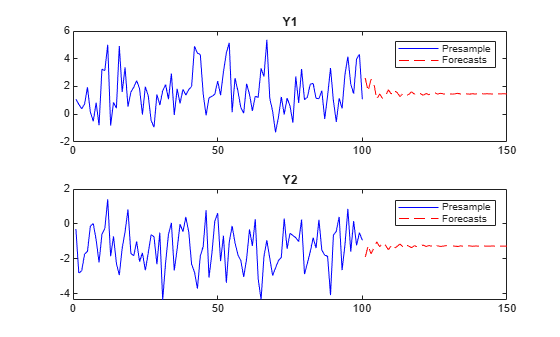Consider including regression components for exogenous variables in each submodel of the threshold-switching dynamic regression model in Forecast Multivariate LSETAR Model.

Fully Specify LSETAR Model

Create logistic threshold transitions at mid-levels -1 and 1, with rates 3.5 and 1.5, respectively. Label the states.

```t = [-1 1]; r = [3.5 1.5]; stateNames = ["Low" "Med" "High"]; tt = threshold(t,Type="logistic",Rates=[3.5 1.5],StateNames=stateNames)```
```tt = threshold with properties: Type: 'logistic' Levels: [-1 1] Rates: [3.5000 1.5000] StateNames: ["Low" "Med" "High"] NumStates: 3 ```

Assume the following VARX models describe the response processes of the system:

• State 1: ${y}_{t}=\left[\begin{array}{c}1\\ -1\end{array}\right]+\left[\begin{array}{c}1\\ -1\end{array}\right]{x}_{1,t}+{\epsilon }_{1,t},$ where ${\epsilon }_{1,t}\sim N\left(\left[\begin{array}{c}0\\ 0\end{array}\right],\left[\begin{array}{cc}1& -0.1\\ -0.1& 1\end{array}\right]\right).$

• State 2: ${y}_{t}=\left[\begin{array}{c}2\\ -2\end{array}\right]+\left[\begin{array}{cc}2& 2\\ -2& -2\end{array}\right]{x}_{2,t}+\left[\begin{array}{cc}0.5& 0.1\\ 0.5& 0.5\end{array}\right]{y}_{t-1}+{\epsilon }_{2,t},$ where ${\epsilon }_{2,t}\sim N\left(\left[\begin{array}{c}0\\ 0\end{array}\right],\left[\begin{array}{cc}2& -0.2\\ -0.2& 2\end{array}\right]\right).$

• State 3: ${y}_{t}=\left[\begin{array}{c}3\\ -3\end{array}\right]+\left[\begin{array}{ccc}3& 3& 3\\ -3& -3& -3\end{array}\right]{x}_{3,t}+\left[\begin{array}{cc}0.25& 0\\ 0& 0\end{array}\right]{y}_{t-1}+\left[\begin{array}{cc}0& 0\\ 0.25& 0\end{array}\right]{y}_{t-2}+{\epsilon }_{3,t},$ where ${\epsilon }_{3,t}\sim N\left(\left[\begin{array}{c}0\\ 0\end{array}\right],\left[\begin{array}{cc}3& -0.3\\ -0.3& 3\end{array}\right]\right).$

${\mathit{x}}_{1,\mathit{t}}$ represents a single exogenous variable, ${\mathit{x}}_{2,\mathit{t}}$ represents two exogenous variables, and ${\mathit{x}}_{3,\mathit{t}}$ represents three exogenous variables. Store the submodels in a vector.

```% Constants (numSeries x 1 vectors) C1 = [1; -1]; C2 = [2; -2]; C3 = [3; -3]; % Regression coefficients (numSeries x numRegressors matrices) Beta1 = [1; -1]; % 1 regressor Beta2 = [2 2; -2 -2]; % 2 regressors Beta3 = [3 3 3; -3 -3 -3]; % 3 regressors % Autoregression coefficients (numSeries x numSeries matrices) AR1 = {}; AR2 = {[0.5 0.1; 0.5 0.5]}; AR3 = {[0.25 0; 0 0] [0 0; 0.25 0]}; % Innovations covariances (numSeries x numSeries matrices) Sigma1 = [1 -0.1; -0.1 1]; Sigma2 = [2 -0.2; -0.2 2]; Sigma3 = [3 -0.3; -0.3 3]; % VARX submodels mdl1 = varm(Constant=C1,AR=AR1,Beta=Beta1,Covariance=Sigma1); mdl2 = varm(Constant=C2,AR=AR2,Beta=Beta2,Covariance=Sigma2); mdl3 = varm(Constant=C3,AR=AR3,Beta=Beta3,Covariance=Sigma3); mdl = [mdl1; mdl2; mdl3];```

Create an LSETAR model from the switching mechanism `tt` and the state-specific submodels `mdl`. Label the series `Y1` and `Y2`.

`Mdl = tsVAR(tt,mdl,SeriesNames=["Y1" "Y2"]);`

Forecast Responses Ignoring Regression Component

If you do not supply exogenous data, `forecast` ignores the regression components in the submodels.

Obtain a presample, from which to forecast, by simulating one 2-D path of 100 observations from the model. Specify the endogenous threshold variable and its delay, ${\mathit{y}}_{2,\mathit{t}-4}$.

```rng(1) % For reproducibility numObs = 100; Y0F = simulate(Mdl,numObs,Delay=4,Index=2);```

Forecast the LSETAR model into a 50-period horizon. Specify the endogenous threshold variable and its delay.

```fh = 50; YF = forecast(Mdl,Y0F,fh,Index=2,Delay=4);```

Plot the simulated path and forecasts for each variable on separate plots.

```figure; plot(1:numObs,Y0F(:,1),"b",(numObs+1):(numObs+fh),YF(:,1),"r--") title("Y1") legend(["Presample" "Forecasts"]) nexttile```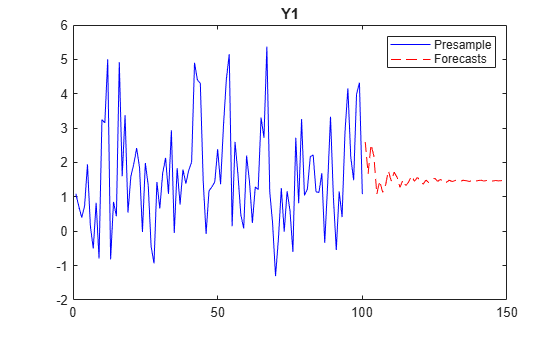```plot(1:numObs,Y0F(:,2),"b",(numObs+1):(numObs+fh),YF(:,2),"r--") legend(["Presample" "Forecasts"]) title("Y2")```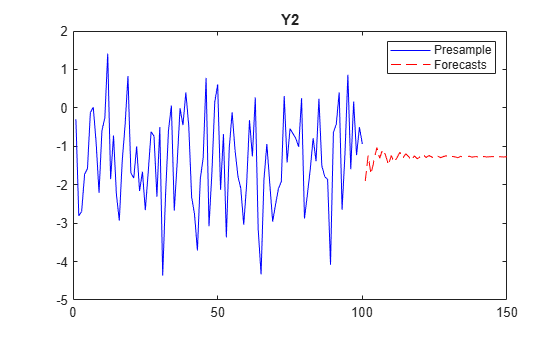Simulate Data Including Regression Component

To generate random paths from the model, `forecast` requires expgenous data.

Assume the following AR models for the regressors:

• ${x}_{1,t}=1+0.1{x}_{1,t-1}+{\nu }_{1,t}$, where ${\nu }_{1,\mathit{t}}\sim Ν\left(0,1\right)$.

• ${x}_{2,t}=2+0.2{x}_{2,t-2}+{\nu }_{2,t}$, where ${\nu }_{2,\mathit{t}}\sim Ν\left(0,2\right)$.

• ${x}_{3,t}=3+0.3{x}_{3,t-3}+{\nu }_{3,t}$, where ${\nu }_{1,\mathit{t}}\sim Ν\left(0,3\right)$.

```MdlX1 = arima(Constant=1,AR={0.1},Variance=1); MdlX2 = arima(Constant=2,AR={0 0.2},Variance=2); MdlX3 = arima(Constant=3,AR={0 0 0.3},Variance=3);```

Simulate 100 observations from each model to represent in-sample data for the exogenous predictors.

```X10F = simulate(MdlX1,numObs); X20F = simulate(MdlX2,numObs); X30F = simulate(MdlX3,numObs); X0F = [X10F X20F X30F];```

Forecast 50 observations from each model to represent exogenous data in the forecast horizon. Specify the simulated in-sample data as a presample.

```X1F = forecast(MdlX1,numObs,Y0=X10F); X2F = forecast(MdlX2,numObs,Y0=X20F); X3F = forecast(MdlX3,numObs,Y0=X30F); XF = [X1F X2F X3F];```

Obtain presample response data, from which to forecast, by simulating one 2-D path of 100 observations from the model. Specify the endogenous threshold variable and its delay, and specify the simulated exogenous data.

`Y0FX = simulate(Mdl,numObs,Delay=4,Index=2,X=X0F);`

Forecast the LSETAR model into a 50-period horizon. Specify the endogenous threshold variable and its delay, and specify the exogenous data in the forecast horizon.

```YFX = forecast(Mdl,Y0FX,fh,Index=2,Delay=4,X=XF); figure; plot(1:numObs,Y0FX(:,1),"b",(numObs+1):(numObs+fh),YFX(:,1),"r--") title("Y1") legend(["Presample" "Forecasts"]) nexttile```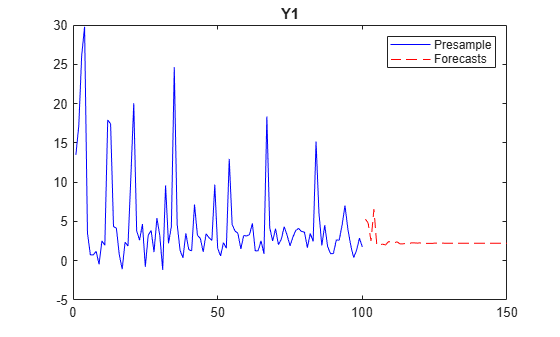```plot(1:numObs,Y0FX(:,2),"b",(numObs+1):(numObs+fh),YFX(:,2),"r--") legend(["Presample" "Forecasts"]) title("Y2")```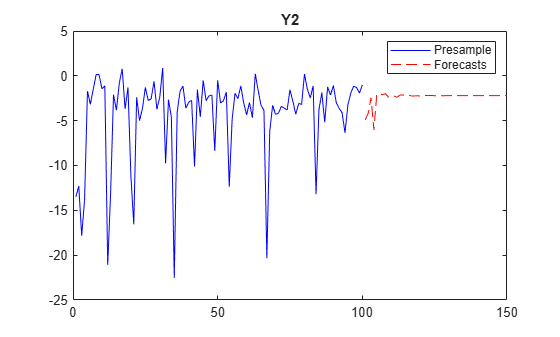## Input Arguments

collapse all

Fully specified threshold-switching dynamic regression model, specified as an `tsVAR` model object returned by `tsVAR` or `estimate`. Properties of a fully specified model object do not contain `NaN` values.

Response data that provides initial values for the forecasts, specified as a `numObs`-by-`numSeries` numeric matrix.

`numObs` is the sample size. `numSeries` is the number of response variables (`Mdl.NumSeries`).

Rows correspond to observations, and the last row contains the latest observation. Columns correspond to individual response variables.

`Y` must contain enough observations to initialize AR terms of all submodels. For self-exciting models, `Y` must also contain enough observations to initialize the delayed response yj,td.

The forecasts `YF` represent the continuation of `Y`.

Data Types: `double`

Forecast horizon, or the number of time points in the forecast period, specified as a positive integer.

Data Types: `double`

### Name-Value Arguments

Specify optional pairs of arguments as `Name1=Value1,...,NameN=ValueN`, where `Name` is the argument name and `Value` is the corresponding value. Name-value arguments must appear after other arguments, but the order of the pairs does not matter.

Before R2021a, use commas to separate each name and value, and enclose `Name` in quotes.

Example: `'X',X` uses the matrix `X` as exogenous data in the forecast horizon to evaluate regression components in the model.

Type of threshold variable data, specified as a value in this table.

ValueDescription
`"endogenous"`

The model is self-exciting with threshold variable data ${z}_{t}={y}_{j,\left(t-d\right)},$ generated by response j, where

`"exogenous"`The threshold variable is exogenous to the system. The name-value argument `'Z'` specifies the threshold variable data and is required.

Example: `Type="exogenous",Z=z` specifies the data `z` for the exogenous threshold variable.

Example: `Type="endogenous",Index=2,Delay=4` specifies the endogenous threshold variable as y2,t−4, whose data is `Y(:,2)`.

Data Types: `char` | `string` | `cell`

Threshold variable data in the forecast horizon, for forecasts of type `"exogenous"`, specified as a numeric vector of length `numPeriods`.

Data Types: `double`

Threshold variable delay d in yj,td, for forecasts of type `"endogenous"`, specified as a positive integer.

Example: `Delay=4` specifies that the threshold variable is y2,td, where j is the value of `Index`.

Data Types: `double`

Threshold variable index j in yj,td, for forecasts of type `"endogenous"`, specified as a scalar in `1:Mdl.NumSeries`.

`forecast` ignores `Index` for univariate AR models.

Example: `Index=2` specifies that the threshold variable is y2,td, where d is the value of `Delay`.

Data Types: `double`

Predictor data in the forecast horizon used to evaluate regression components in all submodels of `Mdl`, specified as a numeric matrix or a cell vector of numeric matrices. The first row of `X` contains observations in the period after the period represented by the last observation in `Y`.

To use a subset of the same predictors in each state, specify `X` as a matrix with `numPreds` columns and at least `numPeriods` rows. Columns correspond to distinct predictor variables. Submodels use initial columns of the associated matrix, in order, up to the number of submodel predictors. The number of columns in the `Beta` property of `Mdl.SubModels(j)` determines the number of exogenous variables in the regression component of submodel `j`. If the number of rows exceeds `numPeriods`, then `forecast` uses the earliest observations.

To use different predictors in each state, specify a cell vector of such matrices with length `numStates`.

By default, `forecast` ignores the regression components in `Mdl`.

Data Types: `double`

Number of sample paths to generate for the simulation, specified as a positive integer. If `forecast` returns only `YF`, it ignores `NumPaths`.

Example: `NumPaths=1000`

Data Types: `double`

## Output Arguments

collapse all

Point forecasts, returned as a `numPeriods`-by-`numSeries` numeric matrix.

If `forecast` returns only `YF`, represents stepwise-optimal forecasts. Otherwise, `forecast` uses Monte Carlo simulation to estimate `YF`.

Forecast error covariances, returned as a numeric column vector or numeric array.

If the submodels `Mdl.SubModels` represent univariate ARX models, `EstCov` is a `numPeriods`-by-1 vector. If `Mdl.SubModels` represent multivariate VARX models, `EstCov` is a `numSeries`-by-`numSeries`-by-`numPeriods` array.

`forecast` performs Monte Carlo simulation to compute `EstCov`.

Note

• Forecast methods for STAR models are discussed in .

• One-step forecasts are minimum-mean-squared-error optimal, but they can become biased over extended horizons .

• Simulation-based forecasts often perform better for extended forecasts , , .

## Tips

 Brown, Bryan W., and Roberto S. Mariano. "Predictors in Dynamic Nonlinear Models: Large-Sample Behavior." Econometric Theory, 5 (December 1989): 430–52. https://doi.org/10.1017/S0266466600012603.

 Clements, Michael P., and Jeremy Smith. "The Performance of Alternative Forecasting Methods for SETAR Models." International Journal of Forecasting, 13 (December 1997): 463–75. https://doi.org/10.1016/S0169-2070(97)00017-4.

 Hyndman, Rob J. "Highest-Density Forecast Regions for Nonlinear and Non-Normal Time Series Models." Journal of Forecasting, 14 (September 1995): 431–41. https://doi.org/10.1002/for.3980140503.

 Lin, Jin-Lung, and Clive W. J. Granger. "Forecasting from Non-Linear Models in Practice." Journal of Forecasting, 3 (January 1994): 1–9. https://doi.org/10.1002/for.3980130102.

 Teräsvirta, Tima. "Modelling Economic Relationships with Smooth Transition Regressions." In A. Ullahand and D.E.A. Giles (eds.), Handbook of Applied Economic Statistics, 507–552. New York: Marcel Dekker, 1998.

 van Dijk, Dick. Smooth Transition Models: Extensions and Outlier Robust Inference. Rotterdam, Netherlands: Tinbergen Institute Research Series, 1999.